Math resources Algebra Math equations

Solve equations with fractions

# Solve equations with fractions

Here you will learn about how to solve equations with fractions, including solving equations with one or more operations. You will also learn about solving equations with fractions where the unknown is the denominator of a fraction.

Students will first learn how to solve equations with fractions in 7th grade as part of their work with expressions and equations and expand that knowledge in 8th grade.

## What are equations with fractions?

Equations with fractions involve solving equations where the unknown variable is part of the numerator and/or denominator of a fraction.

The numerator (top number) in a fraction is divided by the denominator (bottom number).

To solve equations with fractions, you will use the “balancing method” to apply the inverse operation to both sides of the equation in order to work out the value of the unknown variable.

The inverse operation of addition is subtraction.

The inverse operation of subtraction is addition.

The inverse operation of multiplication is division.

The inverse operation of division is multiplication.

For example,

\begin{aligned} \cfrac{2x+3}{5} \, &= 7\\ \colorbox{#cec8ef}{$\times \, 5$} \; & \;\; \colorbox{#cec8ef}{$\times \, 5$} \\\\ 2x+3&=35 \\ \colorbox{#cec8ef}{$-\,3$} \; & \;\; \colorbox{#cec8ef}{$- \, 3$} \\\\ 2x & = 32 \\ \colorbox{#cec8ef}{$\div \, 2$} & \; \; \; \colorbox{#cec8ef}{$\div \, 2$}\\\\ x & = 16 \end{aligned}

### What are equations with fractions?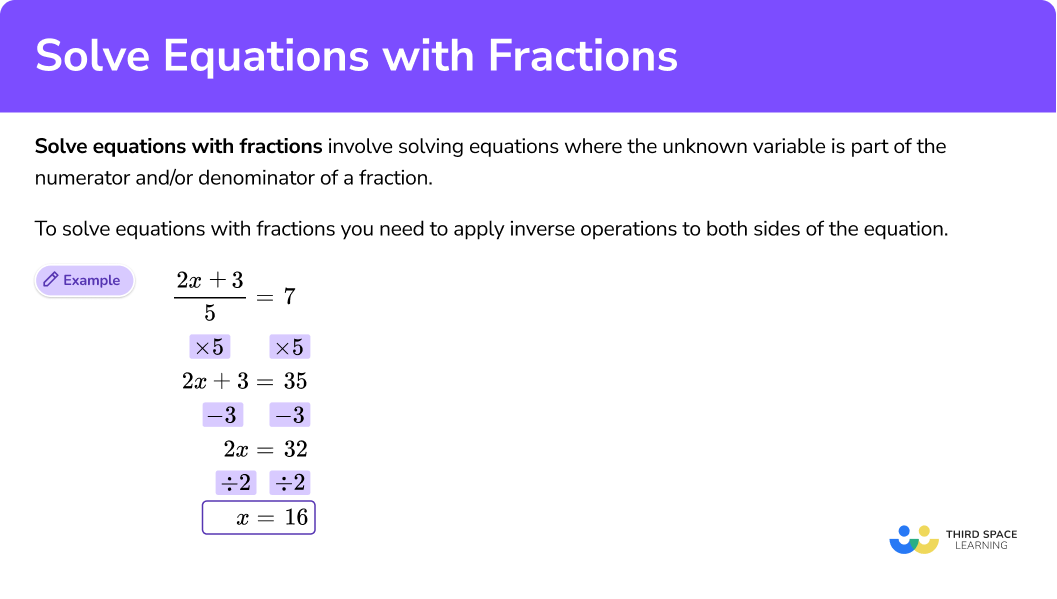## Common Core State Standards

• Grade 7: Expressions and Equations (7.EE.A.1)
Apply properties of operations as strategies to add, subtract, factor, and expand linear expressions with rational coefficients.

• Grade 8: Expressions and Equations (8.EE.C.7)
Solve linear equations in one variable.

• Grade 8: Expressions and Equations (8.EE.C.7b)
Solve linear equations with rational number coefficients, including equations whose solutions require expanding expressions using the distributive property and collecting like terms.

## How to solve equations with fractions

In order to solve equations with fractions:

1. Identify the operations that are being applied to the unknown variable.
2. Apply the inverse operations, one at a time, to both sides of the equation.
3. Write the final answer, checking that it is correct.

## Solve equations with fractions examples

### Example 1: equations with one operation

Solve for x \text{: } \cfrac{x}{5}=4 .

1. Identify the operations that are being applied to the unknown variable.

The unknown is x.

Looking at the left hand side of the equation, the x is divided by 5.

\cfrac{x}{5}

2Apply the inverse operations, one at a time, to both sides of the equation.

The inverse of “dividing by 5 ” is “multiplying by 5 ”.

You will multiply both sides of the equation by 5.

3Write the final answer, checking that it is correct.

You can check the answer by substituting the answer back into the original equation.

\cfrac{20}{5}=20\div5=4

### Example 2: equations with one operation

Solve for x \text{: } \cfrac{x}{3}=8 .

The unknown is x.

Looking at the left hand side of the equation, the x is divided by 3.

\cfrac{x}{3}

The inverse of “dividing by 3 ” is “multiplying by 3 ”.

You will multiply both sides of the equation by 3.

You can check the answer by substituting the answer back into the original equation.

\cfrac{24}{3}=24\div3=8

### Example 3: equations with two operations

Solve for x \text{: } \cfrac{x \, + \, 1}{2}=7 .

The unknown is x.

Looking at the left hand side of the equation, 1 is added to x and then divided by 2 (the denominator of the fraction).

\cfrac{x \, + \, 1}{2}

First, clear the fraction by multiplying both sides of the equation by 2.

Then, subtract 1 from both sides.

You can check the answer by substituting the answer back into the original equation.

\cfrac{13 \, +1 \, }{2}=\cfrac{14}{2}=14\div2=7

### Example 4: equations with two operations

Solve for x \text{: } \cfrac{x}{4}-2=3 .

The unknown is x.

Looking at the left hand side of the equation, x is divided by 4 and then 2 is subtracted.

\cfrac{x}{4}-2

First, add 2 to both sides of the equation.

Then, multiply both sides of the equation by 4.

You can check the answer by substituting the answer back into the original equation.

\cfrac{20}{4}-2=20\div4-2=5-2=3

### Example 5: equations with three operations

Solve for x \text{: } \cfrac{3x}{5}+1=7 .

The unknown is x.

Looking at the left hand side of the equation, x is multiplied by 3, then divided by 5 , and then 1 is added.

\cfrac{3x}{5}+1

First, subtract 1 from both sides of the equation.

Then, multiply both sides of the equation by 5.

Finally, divide both sides by 3.

You can check the answer by substituting the answer back into the original equation.

\cfrac{3 \, \times \, 10}{5}+1=\cfrac{30}{5}+1=6+1=7

### Example 6: equations with three operations

Solve for x \text{: } \cfrac{2x-1}{7}=3 .

The unknown is x.

Looking at the left hand side of the equation, x is multiplied by 2, then 1 is subtracted, and the last operation is divided by 7 (the denominator).

\cfrac{2x-1}{7}

First, multiply both sides of the equation by 7.

Next, add 1 to both sides.

Finally, divide both sides by 3.

You can check the answer by substituting the answer back into the original equation.

\cfrac{2 \, \times \, 11-1}{7}=\cfrac{22-1}{7}=\cfrac{21}{7}=3

### Example 7: equations with the unknown as the denominator

Solve for x \text{: } \cfrac{24}{x}=6 .

The unknown is x.

Looking at the left hand side of the equation, x is the denominator. 24 is divided by x.

\cfrac{24}{x}

You need to multiply both sides of the equation by x.

Then, you can divide both sides by 6.

You can check the answer by substituting the answer back into the original equation.

\cfrac{24}{4}=24\div4=6

### Example 8: equations with the unknown as the denominator

Solve for x \text{: } \cfrac{18}{x}-6=3 .

The unknown is x.

Looking at the left hand side of the equation, x is the denominator. 18 is divided by x , and then 6 is subtracted.

\cfrac{18}{x}-6

First, add 6 to both sides of the equation.

Then, multiply both sides of the equation by x.

Finally, divide both sides by 9.

You can check the answer by substituting the answer back into the original equation.

\cfrac{18}{2}-6=9-6=3

### Teaching tips for solving equations with fractions

• When students first start working through practice problems and word problems, provide step-by-step instructions to assist them with solving linear equations.

• Introduce solving equations with fractions with one-step problems, then two-step problems, before introducing multi-step problems.

• Students will need lots of practice with solving linear equations. These standards provide the foundation for work with future linear equations in Algebra I and II.

• Provide opportunities for students to explain their thinking through writing. Ensure that they are using key vocabulary, such as, absolute value, coefficient, equation, common factors, inequalities, simplify, etc.

### Easy mistakes to make

• The solution to an equation can be any type of number
The unknowns do not have to be integers (whole numbers and their negative opposites). The solutions can be fractions or decimals. They can also be positive or negative numbers.

• The unknown of an equation can be on either side of the equation
The unknown, represented by a letter, is often on the left hand side of the equations; however, it doesn’t have to be. It could also be on the right hand side of an equation.

• When multiplying both sides of an equation, multiply each and every term
When multiplying each side of the equation by a number, it is a common mistake to forget to multiply every term.
For example,
Solve:
\cfrac{x}{2}+3=9

Here, the + 3 was not multiplied by 2, resulting in the incorrect answer.

This person has correctly multiplied each term by the denominator.

• Lowest common denominator (LCD)
It is common to get confused between solving equations involving fractions and adding and subtracting fractions. When adding and subtracting, you need to work out the lowest/least common denominator (sometimes called the least common multiple or LCM). When you solve equations involving fractions, multiply both sides of the equation by the denominator of the fraction.

### Practice solve equations with fractions questions

1. Solve: \cfrac{x}{6}=3

x=9x=36x=12x=18You will multiply both sides of the equation by 6, because the inverse of “dividing by 6 ” is “multiplying by 6 ”.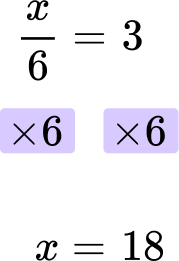The final answer is x = 18.

You can check the answer by substituting the answer back into the original equation.

\cfrac{18}{6}=18 \div 6=3

2. Solve: \cfrac{x \, + \, 4}{2}=7

x=18x=10x=26x=30First, clear the fraction by multiplying both sides of the equation by 2.

Then subtract 4 from both sides.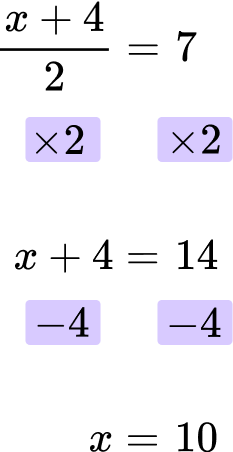The final answer is x = 10.

You can check the answer by substituting the answer back into the original equation.

\cfrac{10 \, + \, 4}{2}=\cfrac{14}{2}=14 \div 2=7

3. Solve: \cfrac{x}{8}-5=1

x=40x=64x=48x=56First, add 5 to both sides of the equation.

Then multiply both sides of the equation by 8.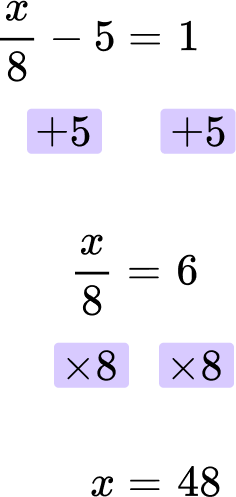The final answer is x = 48.

You can check the answer by substituting the answer back into the original equation.

\cfrac{48}{8}-5=48 \div 8-5=1

4. Solve: \cfrac{3x \, + \, 2}{4}=2

x=4x=16x=12x=2First, multiply both sides of the equation by 4.

Next, subtract 2 from both sides.

Finally, divide both sides by 3.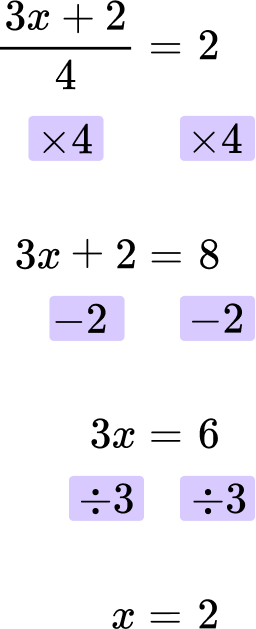The final answer is x = 2.

You can check the answer by substituting the answer back into the original equation.

\cfrac{3 \, \times \, 2+2}{4}=\cfrac{6 \, + \, 2}{4}=\cfrac{8}{4}=8 \div 4=2

5. Solve: \cfrac{4x}{7}-2=6

x=11x=14x=7x=10First, add 2 to both sides of the equation.

Then multiply both sides of the equation by 7.

Finally, divide both sides by 4.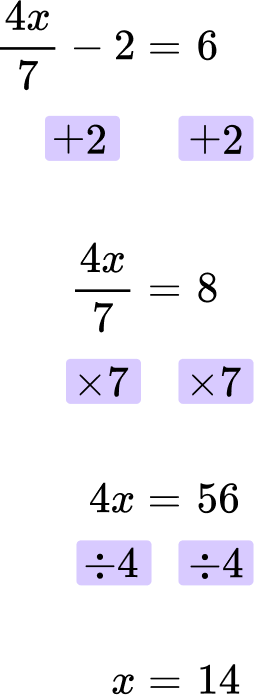The final answer is x = 14.

You can check the answer by substituting the answer back into the original equation.

\cfrac{4 \, \times \, 14}{7}-2=\cfrac{56}{7}-2=56 \div 7-2=6

6. Solve: \cfrac{42}{x}=7

x=5x=294x=7x=6You need to multiply both sides of the equation by x.

Then you divide both sides by 7.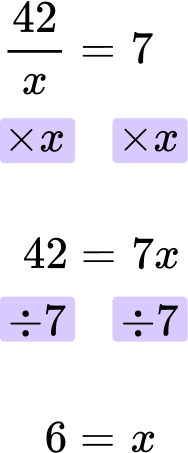The final answer is x = 6.

You can check the answer by substituting the answer back into the original equation.

\cfrac{42}{6}=42 \div 6=7

## Solve equations with fractions FAQs

Do I still follow the order of operations when solving equations with fractions?

Yes, you still follow the order of operations when solving equations with fractions. You will start with any operations in the numerator and follow PEMDAS (parenthesis, exponents, multiply/divide, add/subtract), followed by any operations in the denominator. Then you will solve the rest of the equation as usual.

## Still stuck?

At Third Space Learning, we specialize in helping teachers and school leaders to provide personalized math support for more of their students through high-quality, online one-on-one math tutoring delivered by subject experts.

Each week, our tutors support thousands of students who are at risk of not meeting their grade-level expectations, and help accelerate their progress and boost their confidence.